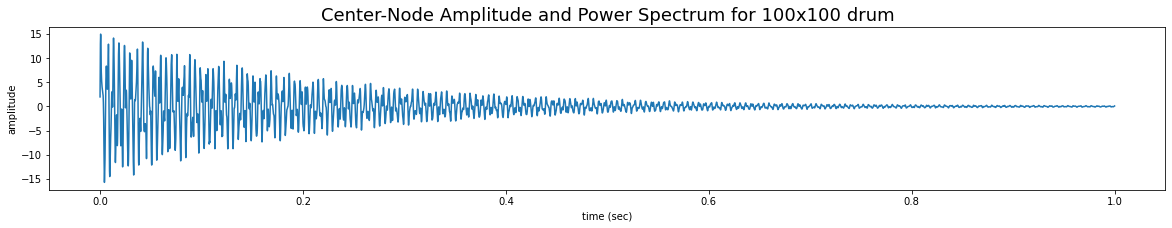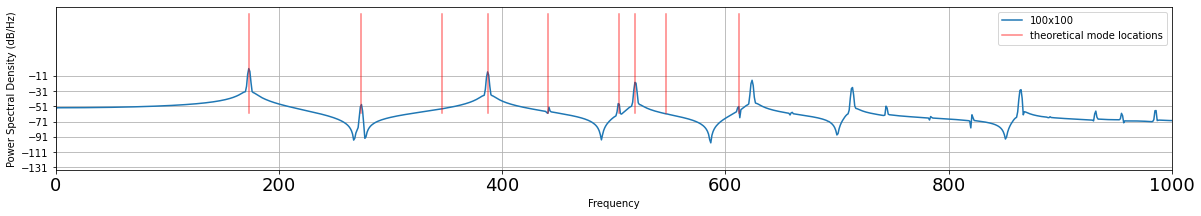# Multiprocessor Drum Synthesis¶

#### ECE 5760, Spring 2023, Adams/Land¶

In :
from scipy.io import wavfile
from IPython.display import Audio
import numpy
from scipy.fft import fft
from scipy.signal import welch
import matplotlib.pyplot as plt
from IPython.display import HTML
plt.rcParams["figure.figsize"] = (20,3)
%matplotlib inline


In :
HTML('''<script>
code_show=true;
function code_toggle() {
if (code_show){
$('div.input').hide(); } else {$('div.input').show();
}
code_show = !code_show
}
$( document ).ready(code_toggle); </script> <form action="javascript:code_toggle()"><input type="submit" value="Click here to toggle on/off the raw code."></form>''')  Out: ## Introduction¶ In this lab, you are challenged to use the hardware resources on the FPGA as wisely as you can to accelerate a simulation of the 2D wave equation on a square mesh. The amplitude of the center node in the mesh will be communicated to the DAC at 48kHz to produce drum-like sounds. Your simulation will include a nonlinear effect related to the instantaneous tension in the mesh that produces the pitch-glide heard in real drums. You are challenged to simulate the largest drum that you can. The group that uses the DSP blocks and memory blocks on the FPGA most exhaustively (as few as possible left over) and efficiently (as many as possible being used in parallel) will be able to produce the largest drum. Last year, the winning group's drum contained over 88,000 nodes! You will be asked to compare the sizes of the largest drums that you can simulate using only the HPS vs. the FPGA. This lab will give you a sense of the FPGA's power for hardware acceleration. ## Mathematical Background¶ A wave equation relates time derivatives to spatial derivates. The continuous form of the 2D wave equation is given below, where$c$is the speed of sound and$uis the out-of-plane amplitude of the node. \begin{align} \frac{1}{c^2} \frac{\partial^2{u}}{\partial{t}^2} = \frac{\partial^2{u}}{\partial{x^2}} + \frac{\partial^2{u}}{\partial{y^2}} - \eta \frac{\partial{u}}{\partial{t}} \end{align} In order to implement this equation on the FPGA, we will used the discretized version shown below. For a derivation of the below equation from the above equation, see this webpage. The amplitude of a particular node,u_{i,j}$at the next timestep,$n+1$, is a function of the node's amplitude at the current and previous timesteps ($n$and$n-1) and the amplitudes of the four adjacent nodes at the current timestep. \begin{align} u_{i,j}^{n+1} = \left[1 + \frac{\eta \Delta t}{2}\right]^{-1}\left\{ \rho_{eff}\left[ u_{i+1,j}^n + u_{i-1,j}^n + u_{i,j-1}^n + u_{i,j+1}^n - 4u_{i,j}^n\right] + 2u_{i,j}^n - \left[1 - \frac{\eta \Delta t}{2}\right]u_{i,j}^{n-1}\right\} \end{align} (Take a close look at that first term. If\frac{\eta \Delta t}{2}$is small, is there an opportunity for simplification?) All of the other terms in the above expression are constants, except for$\rho_{eff}\$. The value for this term is nonlinearly related to the amplitude of the center node according to the equation given below. This nonlinear coupling generates an audible "pitch-glide" just like is heard in real drums. In order for the drum to be stable, this value must be less than 0.5.

\begin{align} \rho_{eff} = \text{min}\left(0.49, \rho_0 +[u_{center} \cdot G_{tension}]^2\right) \end{align}

Reasonable values for the other parameters might be

\begin{align} \rho_0 &\approx 0.25\\ G_{tension} &\approx 2^{-3} \rightarrow 2^{-5}\\ \frac{\eta \Delta t}{2}&\approx 2^{-9} \rightarrow 2^{-10} \end{align}

#### Some sample code¶

• Matlab program from Bruce gives a sequential version of the algorithm and plots the Fourier modes of the drum.
• Clicking "show code" at the top of this webpage will reveal Python code by Hunter based on Bruce's Matlab code (for the more Pythonically inclined person). Like the Matlab, it gives a sequential version of the algorithm and plots the Fourier modes of the drum. Plots given below. It compares the simulated and theoretical drum modes, as given in Physical Modeling with the 2-D Digital Waveguide Mesh.
• Bruce's HPS code which implements the above equation in C for realtime synthesis on the HPS
• If you play with the boundary conditions, damping, wave speed, drum size, and initial conditions, the sound will be drum-like, chime-like, gong-like, or bell-like.
In :
# Initializize drum
n = 100 ;
u = numpy.zeros((n,n));
u1 = numpy.zeros((n,n));
u2 = numpy.zeros((n,n));
uHit = numpy.zeros((n,n));

# Set rho, sample rate, and simulation time
rho = 0.25;
Fs = 48000 ;
time = numpy.arange(0, 1., (1./Fs))

# Array in which to store audio samples
aud = numpy.zeros(len(time)) ;

# Compute middle nodes
x_mid = int(n/2.);
y_mid = int(n/2.);

# Initialize drum amplitudes to a pyramid
for i in range(1, n-1):
for j in range(1,n-1):
uHit[i,j] = max(0, 30-(abs(x_mid - i) + abs(y_mid - j)))/30.;

# Zero boundary conditions
uHit[0,:] = 0
uHit[n-1,:] = 0
uHit[:,0] = 0
uHit[:,n-1] = 0

# Indexing variable
tindex = 0;

# Loop through time array
for t in time:

u = numpy.zeros((n,n))

# Hit the drum at t=0
if (t==0):
u1 = u1 + uHit;

# Compute updated drum amplitudes
u[1:n-2,1:n-2] = (1./(1+.0001)) * (rho * (u1[2:n-1,1:n-2]+
u1[0:n-3,1:n-2]+
u1[1:n-2,2:n-1]+
u1[1:n-2,0:n-3]-
4*u1[1:n-2,1:n-2])
+ 2*u1[1:n-2,1:n-2]
- (1-.0001) * (u2[1:n-2,1:n-2]) ) ;

# Amplitude of center node goes into audio array
aud[tindex] = u[n//2,n//2] ;

# Update/store drum state
u2 = u1;
u1 = u;

# Iterate
tindex = tindex + 1;

# Plot the center node amplitudes
plt.plot(time, aud)
plt.xlabel('time (sec)')
plt.ylabel('amplitude')
plt.title('Center-Node Amplitude and Power Spectrum for 100x100 drum', fontsize=18)
plt.figsize=(20,3)
plt.show()

# Plot the power spectrum
plt.rcParams["figure.figsize"] = (20,3)
Pxxt, freqst = plt.psd(aud,
NFFT=len(aud),
Fs=48000,
window=numpy.hamming(len(aud)),
noverlap=50, label='100x100')

# Plot the theoretical mode locations
fundamental = 173
yvals = numpy.linspace(-60, 70)
modes = [1.58, 2.0, 2.24, 2.55, 2.92, 3.0, 3.16, 3.54]
plt.plot(numpy.ones(len(yvals))*fundamental,
yvals,
'red',
alpha=0.5, label='theoretical mode locations')
for i in modes:
plt.plot(numpy.ones(len(yvals))*fundamental*i,
yvals,
'red',
alpha=0.5)

# Configure plots
plt.xlim([0,1000])
plt.xticks(size=18)
plt.legend(loc='best')
plt.show()

# Output audio
print("Audio - Python synthesized, 100x100 drum")
Audio(aud, rate=48000)Audio - Python synthesized, 100x100 drum

Out:
In :
# Initializize drum
n = 100 ;
u = numpy.zeros((n,n));
u1 = numpy.zeros((n,n));
u2 = numpy.zeros((n,n));
uHit = numpy.zeros((n,n));

# Set rho, sample rate, and simulation time
rho = 0.25;
rho_eff = 0.25;
Fs = 48000 ;
time = numpy.arange(0, 1, (1./Fs))

# Array in which to store audio samples
aud = numpy.zeros(len(time)) ;

# Compute middle nodes
x_mid = int(n/2.);
y_mid = int(n/2.);

# Initialize drum amplitudes to a pyramid
for i in range(1, n-1):
for j in range(1,n-1):
uHit[i,j] = max(0, 30-(abs(x_mid - i) + abs(y_mid - j)))/30.;

# Zero boundary conditions
uHit[0,:] = 0
uHit[n-1,:] = 0
uHit[:,0] = 0
uHit[:,n-1] = 0

# Indexing variable
tindex = 0;

# Loop through time array
for t in time:

u = numpy.zeros((n,n))

# Hit the drum at t=0
if (t==0):
u1 = u1 + uHit;

# Compute updated drum amplitudes
u[1:n-2,1:n-2] = (1./(1+.0001)) * (rho_eff * (u1[2:n-1,1:n-2]+
u1[0:n-3,1:n-2]+
u1[1:n-2,2:n-1]+
u1[1:n-2,0:n-3]-
4*u1[1:n-2,1:n-2])
+ 2*u1[1:n-2,1:n-2]
- (1-.0001) * (u2[1:n-2,1:n-2]) ) ;

# Amplitude of center node goes into audio array
aud[tindex] = u[n//2,n//2] ;

# Update/store drum state
u2 = u1;
u1 = u;

# Update rho
rho_eff = min(0.49, rho + ((1./16)*u[n//2,n//2])**2.)

# Iterate
tindex = tindex + 1;

# Output audio
print("Audio - Python synthesized, nonlinear (exaggerated) rho, 100x100 drum")
Audio(aud, rate=48000)

Audio - Python synthesized, nonlinear (exaggerated) rho, 100x100 drum

Out:

#### Examples of FPGA-synthesized drums¶

Sound from a 170x50 drum

In :
drum = numpy.array([float(i+i)/2. for i in data[110000:160000]])
drum = drum - numpy.mean(drum)
Audio(drum, rate=samplerate)

Out:

Sound from a 170x100 drum

In :
drum3 = numpy.array([float(i+i)/2. for i in data3[120000:180000]])
drum3 = drum3 - numpy.mean(drum3)
Audio(drum3, rate=samplerate3)

Out:

Sound from a linear drum (170x170)

In :
drum_linear = numpy.array([float(i+i)/2. for i in data_linear[100000:160000]])
drum_linear = drum_linear - numpy.mean(drum_linear)
Audio(drum_linear, rate=samplerate_linear)

Out: